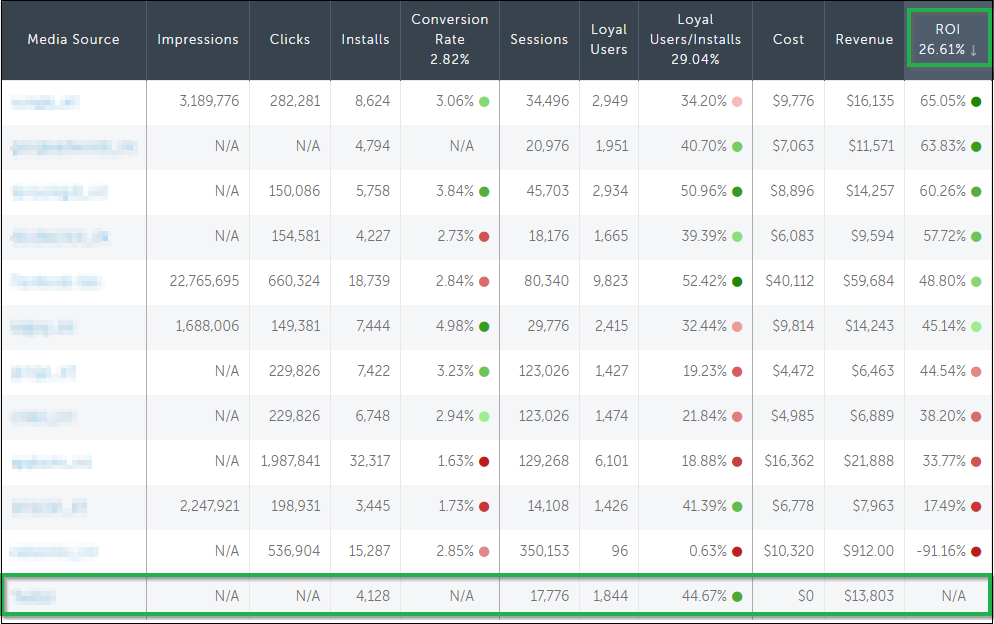# Cost sharing for ad networks

## Why share cost?

Advertisers use AppsFlyer’s dashboard as their source of truth for all budget allocation decisions, making it easy to see where to invest. For networks wanting to be visible in that process, sharing cost data with AppsFlyer is an absolute must.

Advertisers often check their top performing partners using sort by ROI. Those with no cost data always appear at the bottom of the list.## How to share cost?

There are two ways to pass user-level cost data through AppsFlyer attribution urls: unencrypted and encrypted. Encrypted cost pass allows partners to send cost data in the impression and/or click with displaying the values in plain text.

Unencrypted cost can be passed by hardcoding the following 3 parameters to the click URL:

• af_cost_model=CPI (currently CPI is the only model viewable in AppsFlyer reporting)
• af_cost_ value={insert CPI value, e.g. 1.00}
• af_cost_currency={insert 3 letter currency code. If blank we will assume USD}

NOTE: While any cost model can be sent via the attribution link, only CPI is currently supported for presenting cost data on the dashboard. Other cost models are available in the raw reports.

``````https://app.appsflyer.com/id554782625?pid=mediasource_int&c=campaignName&idfa=ABCDEFGH-ABCD-ABCD-ABCD-
A12345678910&af_siteid=12345&af_cost_model=CPI&af_cost_value=1.00&af_cost_currency=USD``````

Encrypted cost

If you prefer not to reveal the actual cost value in the attribution URL, you can encrypt the cost parameters. The encrypted cost can be passed by following an AES-based encryption method. In addition to cost model, value, and currency, partners will need a network specific key to generate the encrypted cost value.

Steps for encryption:

1. Request your network encryption key from your Partner Development Manager or reach out to partners@appsflyer.com.
2. Use the AES encryption method (shown below) to encrypt the required 4 values (network key; cost value; cost model; and cost currency)
3. Append the generated encryption value to your AppsFlyer attribution URL in the af_enc_data= parameter.

https://app.appsflyer.com/id554782625?pid=mediasource_int&c=campaignName&idfa=ABCDEFGH-ABCD-ABCD-ABCD-A12345678910&af_siteid=12345&af_enc_data=DvVvQRow%2F2N0LiQGZh0wvwucFygbll9IwAMSN%2B%2BDQxo%3D

Note - Only ad networks that perform the cost encryption integration with AppsFlyer can actually send encrypted cost data with their clients' attribution links. These networks have the label of "encrypted click" in the following list of cost supporting ad networks.

## AES encryption process

To accomplish the encryption, partners must use the AES algorithm, with an ECB block mode, using PKCS5 padding. Then they must Base 64 encode the encrypted data, and finally URL encode the result.

Networks can use any tech stack to accomplish this. Our official examples provided by r&d are below in PHP, Java, and Python:

PHP

``````<?php
\$f = fopen( 'php://stdin', 'r' );
echo "Enter private key: ";
\$private_key = trim(fgets(\$f));
echo "Enter cost model: ";
\$cost_model = trim(fgets(\$f));
echo "Enter cost value : ";
\$cost_value = trim(fgets(\$f));
echo "Enter cost currency : ";
\$cost_currency = trim(fgets(\$f));
\$params = array(
'af_cost_model' => \$cost_model,
'af_cost_value' => \$cost_value,
'af_cost_currency' => \$cost_currency
);
\$query_str = http_build_query(\$params);
\$block_size = mcrypt_get_block_size(MCRYPT_RIJNDAEL_128, MCRYPT_MODE_ECB);
\$padding_size = \$block_size - (strlen(\$query_str) % \$block_size);
\$enc_raw = mcrypt_encrypt(MCRYPT_RIJNDAEL_128, \$private_key, \$padded_query_str, "ecb");
\$enc_b64 = base64_encode(\$enc_raw);
\$enc_url_encoded = urlencode(\$enc_b64);
echo "\n\n\r";
echo "Private key: " . \$private_key . "\r\n";
echo "Query string: " . \$query_str . "\r\n";
echo "\n\r";
echo "Encrypted query string: " . \$enc_url_encoded . "\r\n";
fclose( \$f );
?>``````

JAVA

``````private static final String CIPHER_ALGORITHM = "AES/ECB/PKCS5Padding";
private static final String encryptionKey = "XXXXXXXXXXXXXXXX"; // secret key provided by appsflyer
private static final SecretKeySpec key = new SecretKeySpec(encryptionKey.getBytes(), "AES");
public static void main(String[] args) throws Exception {
String queryParams = "bid_value=0.01&bid_type=cpc";
String cipher = encrypt(queryParams);
System.out.println(cipher);
}
public static String encrypt(String str) throws Exception {
Cipher cipherEncrypt = Cipher.getInstance(CIPHER_ALGORITHM);
cipherEncrypt.init(Cipher.ENCRYPT_MODE, key);
byte[] cipher = cipherEncrypt.doFinal(str.getBytes("UTF-8"));
String b64 = encode64(cipher);
String result = urlencode(b64);
return result;
}``````

PYTHON

``````import sys
import base64
import urllib
from Crypto.Cipher import AES
try:
start = input("enter " + t + ": ")
start = float(start)
return start
except ValueError:
print("\n\r" + t + " must be a number")
exit()
def encrypt (secret_key, msg):
cipher = AES.new(secret_key, AES.MODE_ECB)
ciphertext = cipher.encrypt(msg)
return ciphertext
length = 16 - (len(data) % 16)
data += chr(length)*length
return data

secret_key = input("enter secret key: ").strip()
currency = input("enter cost currency: ").strip()
model = "CPI"
if len(currency) == 3:
currency = currency.upper()
else:
print("currency must have 3 character (e.g. USD)")
exit()
if end >= start:
while start <= end:
msg = "af_cost_currency=%s&af_cost_model=%s&af_cost_value=%.2f" % (currency, model, start)